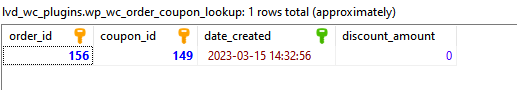### How to compute WooCommerce order count per coupon code

The first thing to do when computing the order count per coupon code in WooCommerce is to determine the actual coupon ID. That’s easily achieved using wc_get_coupon_id_by_code. The function will either return the coupon ID or 0 if not found:

``\$couponId = wc_get_coupon_id_by_code(\$couponCode);``

Next, and the most important step, is using the wc_order_coupon_lookup table to determine the order count for the coupon ID we have just obtained. Of course, we must be sure to add the configured database table prefix to this table name. This table maintains, for each order (based on order ID – order_id column), what coupon has been used (based on coupon ID – coupon_id column).And so, here’s an example:

``````/** @var \wpdb \$wpdb */
global \$wpdb;
\$tableName = \$wpdb->prefix . 'wc_order_coupon_lookup';
\$sql = 'SELECT COUNT(cl.order_id) AS coupon_count
FROM ' . \$tableName . ' cl
WHERE cl.coupon_id = ' . intval(\$couponId) . '
GROUP BY cl.coupon_id';

\$orderCountData = \$wpdb->get_col(\$sql,
0);

if (!empty(\$orderCountData)) {
\$orderCount = intval(\$orderCountData);
} else {
\$orderCount = 0;
}``````